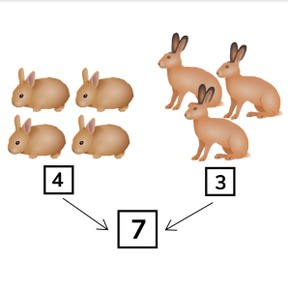Combining numbers to 10

# Combining numbers to 10

Combining numbers to 108,000 schools use Gynzy92,000 teachers use Gynzy1,600,000 students use Gynzy

## General

Students learn to combine numbers to 10. By counting on, or counting fingers, students learn how much the numbers are together.

## Relevance

It is useful to know how much you have together of something. An example is two chickens have laid eggs. You want to know the total of eggs laid, and combine the numbers.

## Introduction

Students first counting disorganized amounts to 10 by counting animal types. Check the answers together on the interactive whiteboard.

## Development

Explain that sometimes it is useful to know what a total number would be. You can combine two numbers to know the total. For example, if you want to know the total number of animals on the farm, you will count and combine the numbers. Explain using the example: 2 cows and 3 pigs combined are 5 animals. It is useful to continue counting. You get, 3-4-5. If you can't do it in your head, you can use your fingers to count on. Show the same with the example of rabbits and hares. Next use number cards to show how to combine numbers without the context of a story or picture. 2 and 6, combined are 8, and 5 and 5 combined are 10. Give students number cards to 10 and ask them to practice combining numbers in pairs. Ask them to hold up the correct number card to answer the combining question on the board.

Check that students understand combining numbers to 10 by asking the following questions:
- Which numbers can combine to make 5?
- What do you get when you combine 3 and 5? How did you do that?

## Guided Practice

Students practice combine numbers by clicking the correct answer or filling in the combined total.

## Closing

Ask students to practice combining numbers to 10 in pairs. One student sets down two cards under 10 and the second student sets down the combined total answer. For example- student 1 sets down 7 and 3, student 2 sets down 10. Discuss that it is useful to know how to combine two numbers so you know what the total is. Ask students to come up with other examples of when it would be useful to know a combined number. Examples: marbles, candies, collectors cards.

## Teaching Tip

Students who have difficulty with combining in their head or on their fingers can practice using the rekenrek or blocks.

## Instruction materials

number cards to 10, rekenrek, blocks

### The online teaching platform for interactive whiteboards and displays in schools

• Save time building lessons

• Manage the classroom more efficiently

• Increase student engagement